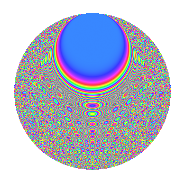# Properties

 Label 1350.2.bbLevel 1350 Weight 2 Character orbit bb Rep. character $$\chi_{1350}(257,\cdot)$$ Character field $$\Q(\zeta_{36})$$ Dimension 648 Sturm bound 540

# Related objects

## Defining parameters

 Level: $$N$$ = $$1350 = 2 \cdot 3^{3} \cdot 5^{2}$$ Weight: $$k$$ = $$2$$ Character orbit: $$[\chi]$$ = 1350.bb (of order $$36$$ and degree $$12$$) Character conductor: $$\operatorname{cond}(\chi)$$ = $$135$$ Character field: $$\Q(\zeta_{36})$$ Sturm bound: $$540$$

## Dimensions

The following table gives the dimensions of various subspaces of $$M_{2}(1350, [\chi])$$.

Total New Old
Modular forms 3384 648 2736
Cusp forms 3096 648 2448
Eisenstein series 288 0 288

## Trace form

 $$648q - 24q^{6} + O(q^{10})$$ $$648q - 24q^{6} - 48q^{11} - 24q^{23} - 12q^{27} + 12q^{33} + 24q^{36} + 36q^{38} + 144q^{41} + 48q^{42} + 48q^{47} + 12q^{48} + 24q^{51} + 24q^{56} + 36q^{57} - 72q^{61} - 84q^{63} + 72q^{67} - 36q^{68} + 48q^{72} + 240q^{77} - 24q^{78} + 48q^{81} + 60q^{83} + 36q^{86} + 252q^{87} + 48q^{92} + 96q^{93} + 72q^{97} + O(q^{100})$$

## Decomposition of $$S_{2}^{\mathrm{new}}(1350, [\chi])$$ into newform subspaces

The newforms in this space have not yet been added to the LMFDB.

## Decomposition of $$S_{2}^{\mathrm{old}}(1350, [\chi])$$ into lower level spaces

$$S_{2}^{\mathrm{old}}(1350, [\chi]) \cong$$ $$S_{2}^{\mathrm{new}}(135, [\chi])$$$$^{\oplus 4}$$$$\oplus$$$$S_{2}^{\mathrm{new}}(270, [\chi])$$$$^{\oplus 2}$$$$\oplus$$$$S_{2}^{\mathrm{new}}(675, [\chi])$$$$^{\oplus 2}$$

## Hecke Characteristic Polynomials

There are no characteristic polynomials of Hecke operators in the database